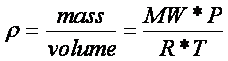The density is defined as the ratio of the mass to the volume.  The density of an ideal gas depends on the molecular weight, temperature and pressure of the system.  Density is an important parameter when performing calculations dealing with heat and mass transfer. It is also used to derive many important dimensionless numbers such as the Reynolds number and the Peclet number.

'Rhocalc' is a matlab based program in start301 that enables the user to calculate the density of an ideal gas after inputting the pressure and temperature for pre-defined compounds.  The constraints on the pressure input dictate that the gas should be ideal and hence the pressure should be less than approximately 10 atmospheres. For pressures greater than this value, non-ideality might become a significant factor and the density value might not be accurate.Here is the matlab source code for rhocalc.

function rhocalc(P,T,index)

global mw % loads molecular weights
global nc %load number of compounds
T=at(T); %converts T to kelvin

%define constants
R=82.0578/(10^3); %gas constant with units of m^3*atm/kg-mol/K

if nargin >2
i=index;

%check to see if all the necessary data is contained in database

if mw(i)==NaN,
fprintf('Molecular weight data for compound *%s* is not available .\n',cnms(i,:))

elseif (T<TbpK(i))
fprintf('The temperature for compound *%s* is below the boiling point.\n',cnms(i,:))

elseif (T<0)
fprintf('The temperature for compound *%s* is below absolute zero,enter a higher temperature.\n',cnms(i,:))

elseif (P> 10)
fprintf('The entered pressure value is too high, the gas may not behave ideally')

elseif (P<0)
fprintf('The entered pressure is below zero,try again')

else %if all data is specified
Rho(i)=mw(i)*P/R/T;
end
Rho(i)
else %if index not specified, calculate data for all compounds

for i=1:nc,
if mw(i)==NaN,
fprintf('Molecular weight data for compound *%s* is not available .\n',cnms(i,:))

elseif (T<TbpK(i))
fprintf('The temperature for compound *%s* is below the boiling point.\n',cnms(i,:))

elseif (T<0)
fprintf('The temperature for compound *%s* is below absolute zero,enter a higher temperature.\n',cnms(i,:))

elseif (P> 10)
fprintf('The entered pressure value is too high, the gas may not behave ideally')

elseif (P<0)
fprintf('The entered pressure is below zero,try again')

else %if all data is specified
Rho(i)=mw(i)*P/R/T;
end

end
Rho
end